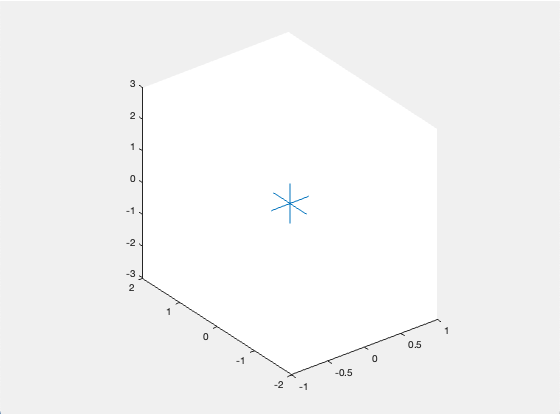File Exchange

## points2axes

version 1.2.0.1 (18.4 KB) by
Conversion factors between points and axis units

Updated 06 May 2021

From GitHub# points2axes

points2axes calculates the conversion factors between points and axis units along the three Cartesian axes.

## Purpose

The conversion factors are useful for drawing objects whose characteristic dimensions such as width, length, height, diameter, etc. are best expressed in points, where 1 point = 1/72 of an inch.

Note that changing the size of the figure window or the limits, orientation, or aspect ratio of the axes directly affects the conversion factors. It is therefore recommended to set the axis limits before calling points2axes to prevent them from changing when new objects are added.

## Usage

`[xppt, yppt, zppt] = points2axes` calculates the conversion factors between points and axis units along the three Cartesian axes for the current axes. `xppt` is the number of x axis units that corresponds to a length of 1 point on the screen. Similarly, `yppt` and `zppt` are the number of y and z axis units per point, respectively.

`[xppt, yppt, zppt] = points2axes(ax)` calculates the conversion factors for the axes specified by `ax` instead of the current axes.

## Limitations

Logarithmic plots of any kind are not supported.

Conversion factors for perspective projections are position-dependent and therefore do not have unique values. In this case, the conversion factors for the corresponding orthographic projection are returned (which gives sensible results in most cases) and a warning is issued.

## Example

```% create figure and axes
figure; ax = axes; ax.Clipping = 'off';
xlim([-1 1]); ylim([-2 2]); zlim([-3 3]);   % set axis limits
view(3); daspect([2 3 5]);                  % set 3D view and data aspect ratio

% calculate conversion factors
[xppt,yppt,zppt] = points2axes;

% draw lines that are 40 points in length
line([-20*xppt 20*xppt], [0 0], [0 0]);
line([0 0], [-20*yppt 20*yppt], [0 0]);
line([0 0], [0 0], [-20*zppt 20*zppt]);```

This creates three 40 point long lines in the center of a 3D plot.## Requirements

points2axes is compatible with MATLAB R2018a and later releases.

## Feedback

Any feedback or suggestions for improvement are welcome!

### Cite As

Jorg Woehl (2021). points2axes (https://github.com/JorgWoehl/points2axes/releases/tag/v1.2.0.1), GitHub. Retrieved .

##### MATLAB Release Compatibility
Created with R2021a
Compatible with R2018a and later releases
##### Platform Compatibility
Windows macOS Linux